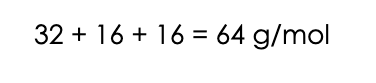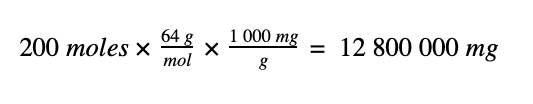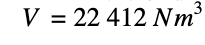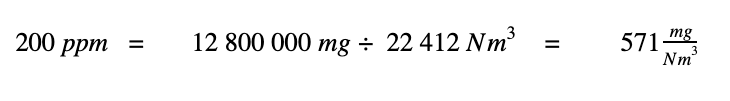Select Page

# Converting from Parts Per Million (ppm) to Milligrams per Normal Cubic Meter (mg/Nm3)

What you need to know

The ability to convert from parts per million (ppm) to milligrams per normal cubic meter (mg/Nm3) is an essential tool in the arsenal of every air quality practitioner. Whether you are interpreting the results of stack testing from a package boiler or an industrial process stack, you will benefit from being able to convert ppm to mg/Nm3.

Isokinetic stack sampling is often not required for gases such as sulphur dioxide (SO2), carbon monoxide (CO), total volatile organic compounds (TVOCs), nitrogen monoxide (NO) and nitrogen dioxide (NO2) [together called “NOx”]. So these pollutants are usually sampled in a non-isokinetic manner, using a gas analyser according to EPA Methods 6c, 7e, 10 and 25b. Most gas analysers report these stack emissions in parts per million.

We need to convert these stack emissions to mg/Nm3, so that we can compare them with the emissions limits that are stated in mg/Nm3 in the legislation. The emissions limits can be found in the listed activities and controlled emitters legislation, which fall under the broader South African legislation that is known as the National Environmental Management Air Quality Act (NEMAQA), Act 39 of 2004.

In the 7 minute video beneath, Caitlin explains from first principles how to convert stack emissions from ppm to mg/Nm3. Having watched the video, please continue reading and then download the pdf at the end so that you have a copy of the table of conversions for your future reference.

As we learnt in the video above, we start with the definition of ppm. A concentration in parts per million describes how many atoms of one component there are, out of one million atoms of the total gas mixture. We can group the atoms into groups called “moles” where 6.02 x 1023 atoms make up one mole. We can just as easily say that ppm is the number of moles of a particular pollutant, out of one million moles of the total gas mixture. Just like one percent would be one in a hundred moles, one ppm is one in a million moles. It is that simple: one percent means one in a hundred; whereas one ppm means one in a million. If you would like to brush up on your understanding of pollutant concentrations, you can read section 2 of the following link, where concentrations are explained simply. So, if we have a pollutant concentration of 200 ppm of sulphur dioxide (SO2) in a total gas mixture that is comprised of SO2 and many other gases such as oxygen, nitrogen, carbon dioxide and more; it simply means that there are 200 moles of SO2 in every one million moles of the total gas mixture. So we can write:To convert from ppm to mg/Nm3 we work with the top and bottom parts of the above formula separately. We start by converting the 200 moles of SO2 to milligrams (mg), and then we convert the 1 000 000 moles of total gas mixture to normal cubic meters (Nm3).   1. Let’s start by converting the 200 moles of the pollutant SO2 to mg. To do this, we need to know the molecular mass of SO2. Sulphur has a mass of 32 g/mol. Oxygen has a mass of 16 g/mol. There are two oxygens in SO2, so the molar mass of SO2 is:To convert the 200 moles of SO2 to a mass in mg we do the following:## 2. To calculate the volume that one million moles of the gas mixture occupies at normal conditions of 0 ˚C (which is the same as 273.15 K) and 101.3 kPa, we can use the ideal gas law as follows:So, from the calculations above: 1. 200 moles of SO2 has a mass of 12 800 000 mg, 2. A million moles of total gas mixture can be stated as a volume of 22 412 Nm3. Dividing these two values leads to the conversion of 200 ppm to units of mg/Nm3.If, instead of using 200 ppm in our calculation above, we simply used 1 ppm, we would discover that 1 ppm of SO2 equals 2.86 mg/Nm3.

If we did the same calculation for CO which has a molar mass of 28 g/mol, we would discover that 1 ppm of CO equals 1.25 mg/Nm3. Likewise we could do the same calculations for NO and NO2. We have done this for you, and beneath is a handy table of conversions. Simply multiply your concentration that is in ppm by the factor and you have the concentration expressed as mg/Nm3. You can now compare the stack emissions that the gas analyser is recording, with the emissions limits that are expressed in mg/Nm3 in the listed activities and controlled emitters legislation.

 Pollutant Conversion Factor (Multiply ppm by this factor to obtain mg/Nm3) Sulphur Dioxide (SO2) 2.86 Carbon Monoxide (CO) 1.25 Nitrogen Monoxide (NO) 1.34 Nitrogen Dioxide (NO2) 2.05 NOx (expressed as NO2) 2.05 Total Volatile Organic Compounds (TVOCs) [expressed as C3H8] 1.96